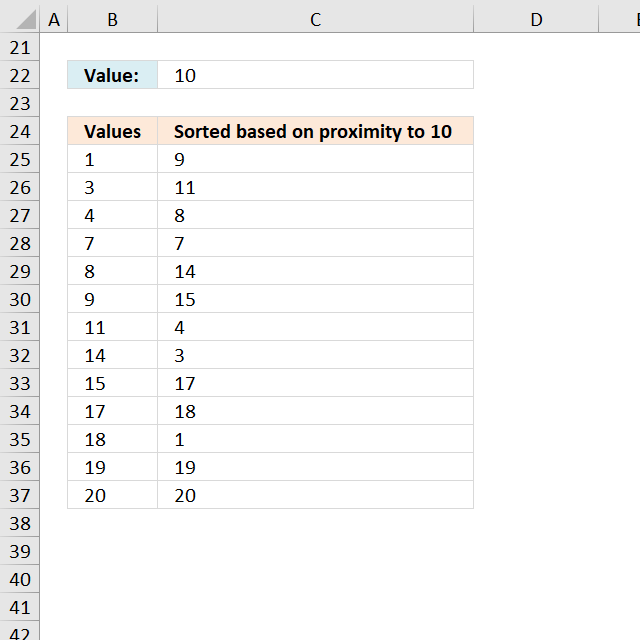Author: Oscar Cronquist Article last updated on December 12, 2018The image above demonstrates an array formula in cell C25 that extracts numbers based on how far off they are from the number in cell C22. Example, 9 and 11 are closest to 10 and are extracted first.

Array formula in C25:

=INDEX(\$B\$25:\$B\$37, MATCH(SMALL(ABS(\$B\$25:\$B\$37-\$C\$22), ROW(A1)), (COUNTIF(\$B\$25:\$B\$37, \$B\$25:\$B\$37)<>COUNTIF(\$D\$24:D24, \$B\$25:\$B\$37))*ABS(\$B\$25:\$B\$37-\$C\$22), 0))

### How to create an array formula

1. Copy (Ctrl + c) and paste (Ctrl + v) array formula into formula bar.
2. Press and hold Ctrl + Shift.
3. Press Enter once.
4. Release all keys.

Copy cell C25 and paste it down as far as needed.

### Explaining array formula

Step 1 - Find k-th smallest difference to the value in C22

=INDEX(\$B\$25:\$B\$37, MATCH(SMALL(ABS(\$B\$25:\$B\$37-\$C\$22);ROW(A1)), (COUNTIF(\$B\$25:\$B\$37, \$B\$25:\$B\$37)<>COUNTIF(\$D\$24:D24, \$B\$25:\$B\$37))*ABS(\$B\$25:\$B\$37-\$C\$22), 0))

SMALL(ABS(\$B\$25:\$B\$37-\$C\$22), ROW(A1))

becomes

SMALL(ABS({1;3;4;7;8;11;11;14;15;17;18;19;20}-10), ROW(A1))

becomes

SMALL({9;7;6;3;2;1;1;4;5;7;8;9;10}, 1)

and returns 1.

Step 2 - Calculate valid numbers

=INDEX(\$B\$25:\$B\$37, MATCH(SMALL(ABS(\$B\$25:\$B\$37-\$C\$22);ROW(A1)), (COUNTIF(\$B\$25:\$B\$37, \$B\$25:\$B\$37)<>COUNTIF(\$D\$24:D24, \$B\$25:\$B\$37))*ABS(\$B\$25:\$B\$37-\$C\$22), 0))

(COUNTIF(\$B\$25:\$B\$37, \$B\$25:\$B\$37)<>COUNTIF(\$D\$24:D24, \$B\$25:\$B\$37))*ABS(\$B\$25:\$B\$37-\$C\$22)

becomes

(COUNTIF({1;3;4;7;8;11;11;14;15;17;18;19;20}, {1;3;4;7;8;11;11;14;15;17;18;19;20})<>COUNTIF("Sorted closest to farthest to 10", {1;3;4;7;8;11;11;14;15;17;18;19;20}))*ABS({1;3;4;7;8;11;11;14;15;17;18;19;20}-10)

becomes

({1;1;1;1;1;2; 2;1;1;1;1;1;1}<>{0;0;0;0;0; 0;0;0;0;0;0;0;0})*ABS({1;3;4;7;8;11;11; 14;15;17;18;19;20}-10)

becomes

({1;1;1;1;1;2; 2;1;1;1;1;1;1}<>{0;0;0;0;0; 0;0;0;0;0;0;0;0})*ABS({1;3;4;7;8;11;11; 14;15;17;18;19;20}-10)

becomes

({TRUE;TRUE;TRUE;TRUE;TRUE; TRUE;TRUE;TRUE;TRUE;TRUE; TRUE;TRUE;TRUE})*{9;7;6;3;2;1;1;4;5;7;8;9;10}

and returns

{9;7;6; 3;2;1; 1;4;5; 7;8;9; 10}

Step 3 - Match k-th smallest difference to valid numbers

=INDEX(\$B\$25:\$B\$37, MATCH(SMALL(ABS(\$B\$25:\$B\$37-\$C\$22);ROW(A1)), (COUNTIF(\$B\$25:\$B\$37, \$B\$25:\$B\$37)<>COUNTIF(\$D\$24:D24, \$B\$25:\$B\$37))*ABS(\$B\$25:\$B\$37-\$C\$22), 0))

MATCH(SMALL(ABS(\$B\$25:\$B\$37-\$C\$22);ROW(A1)), (COUNTIF(\$B\$25:\$B\$37, \$B\$25:\$B\$37)<>COUNTIF(\$D\$24:D24, \$B\$25:\$B\$37))*ABS(\$B\$25:\$B\$37-\$C\$22), 0)

becomes

MATCH(1,{9;7;6; 3;2;1; 1;4;5; 7;8;9; 10}, 0)

returns 6.

Step 4 - Return a value of the cell at the intersection of a particular row and column

=INDEX(\$B\$25:\$B\$37, MATCH(SMALL(ABS(\$B\$25:\$B\$37-\$C\$22);ROW(A1)), (COUNTIF(\$B\$25:\$B\$37, \$B\$25:\$B\$37)<>COUNTIF(\$D\$24:D24, \$B\$25:\$B\$37))*ABS(\$B\$25:\$B\$37-\$C\$22), 0))

becomes

=INDEX(\$B\$25:\$B\$37, 6)

becomes

=INDEX({1;3;4;7;8;11;11;14;15;17;18;19;20}, 6)

returns number 11.

### Get Excel *.xlsx file

Values sorted by diff.xlsx